宏观经济学 第二章（不定时跟新）

1. 支出法 = C+I+G
2. 收入法 = 工资+利息+租金+税前利润+折旧+间接税+企业转移支付
3. 生产法 = 收入-成本

GDP=C+I+G+NX=政府支出+个人支出+企业支出=100000+(20000+20000)+(50000+20000)

下列项目是否计入GDP，为什么？

1.政府转移支付；

2.购买一辆用过的卡车；

3.购买普通股票；

4.购买一块地产。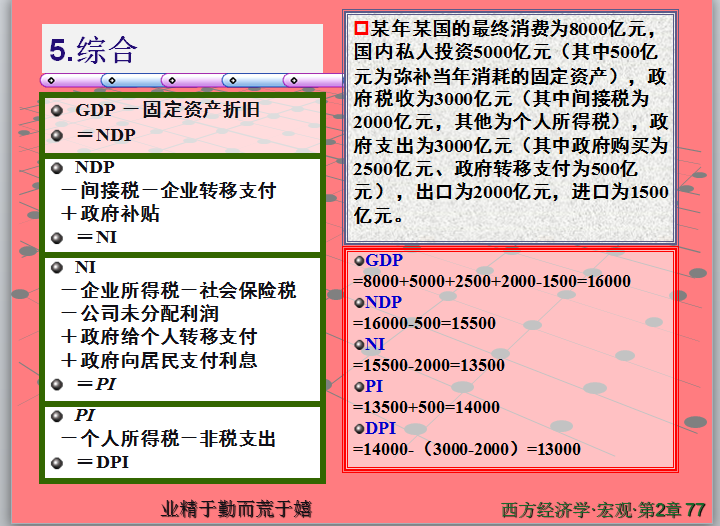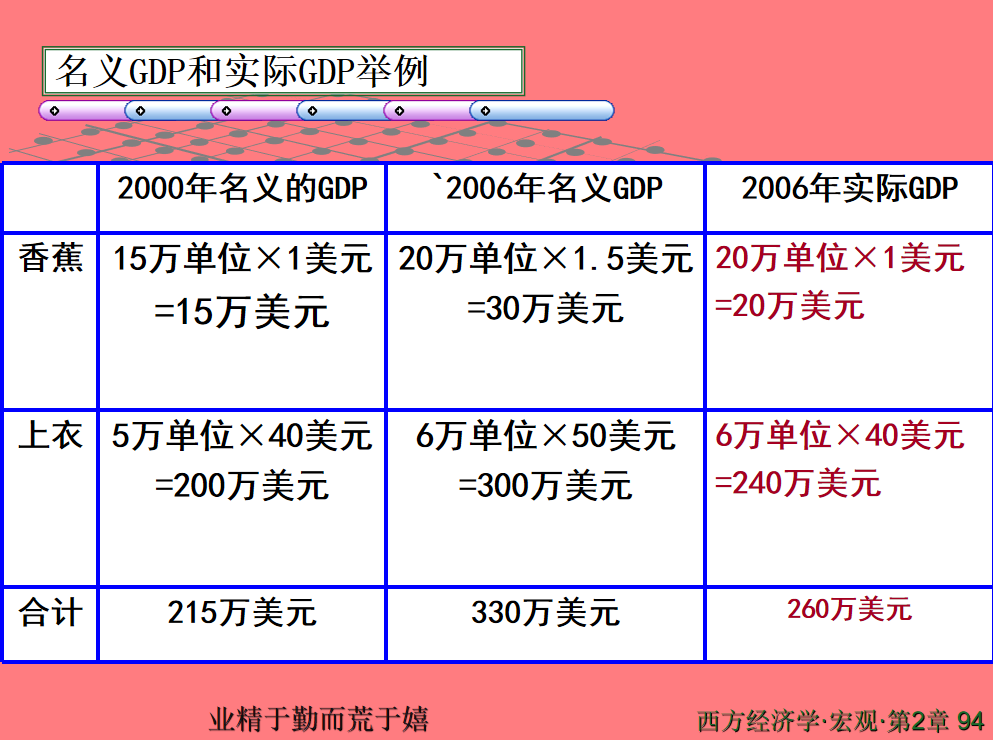CPI：是度量居民生活消费品和服务价格水平随着时间变动的相对数，综合反映居民购买的生活消费品和服务价格水平的变动情况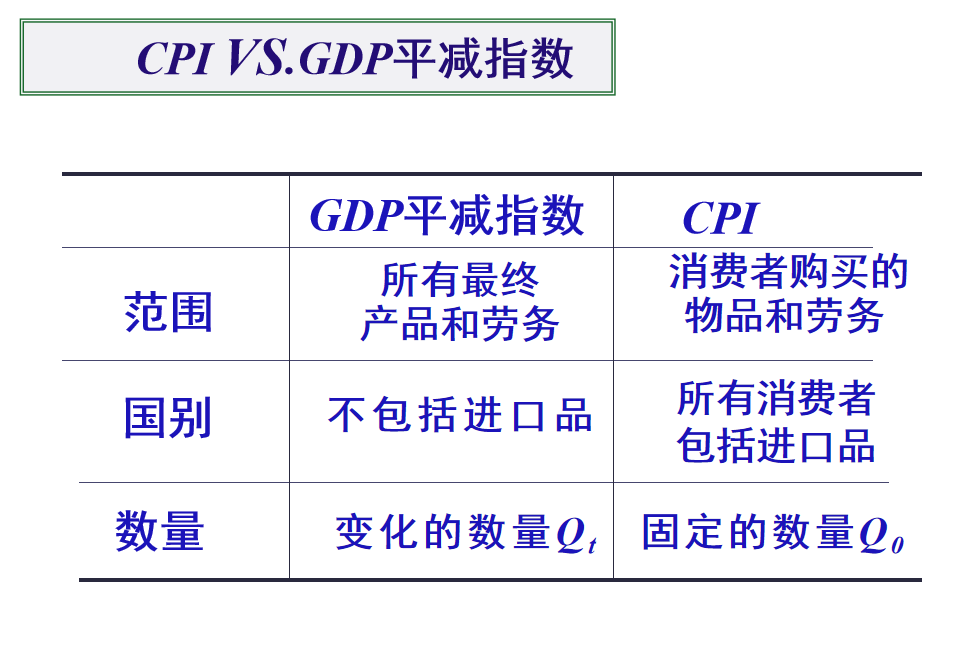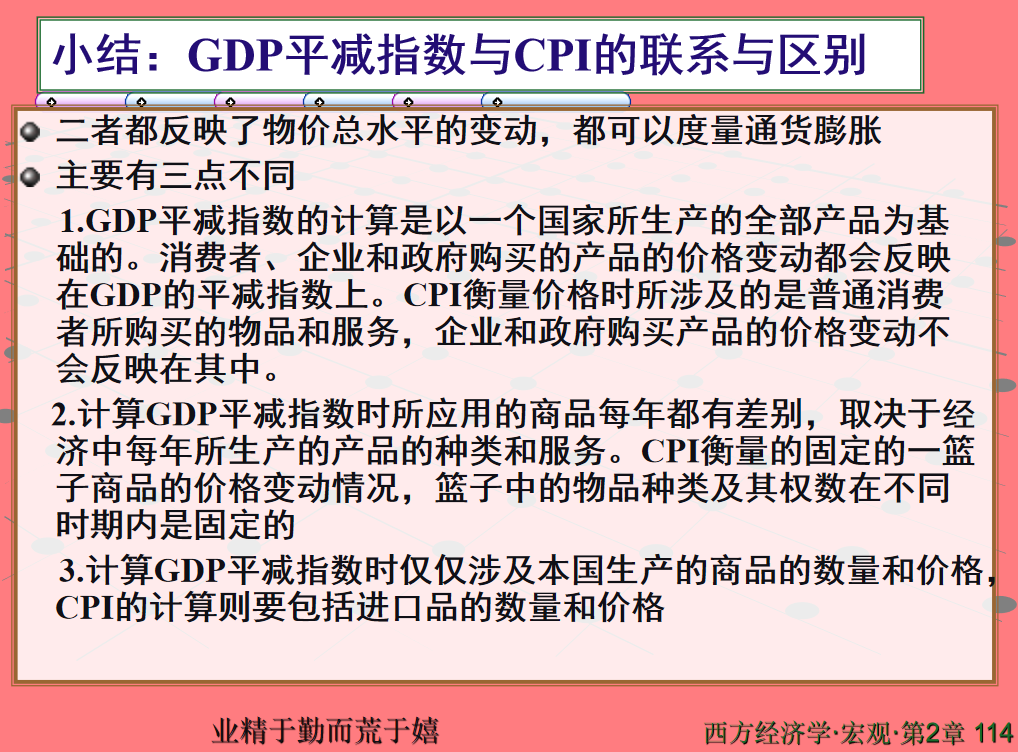练习题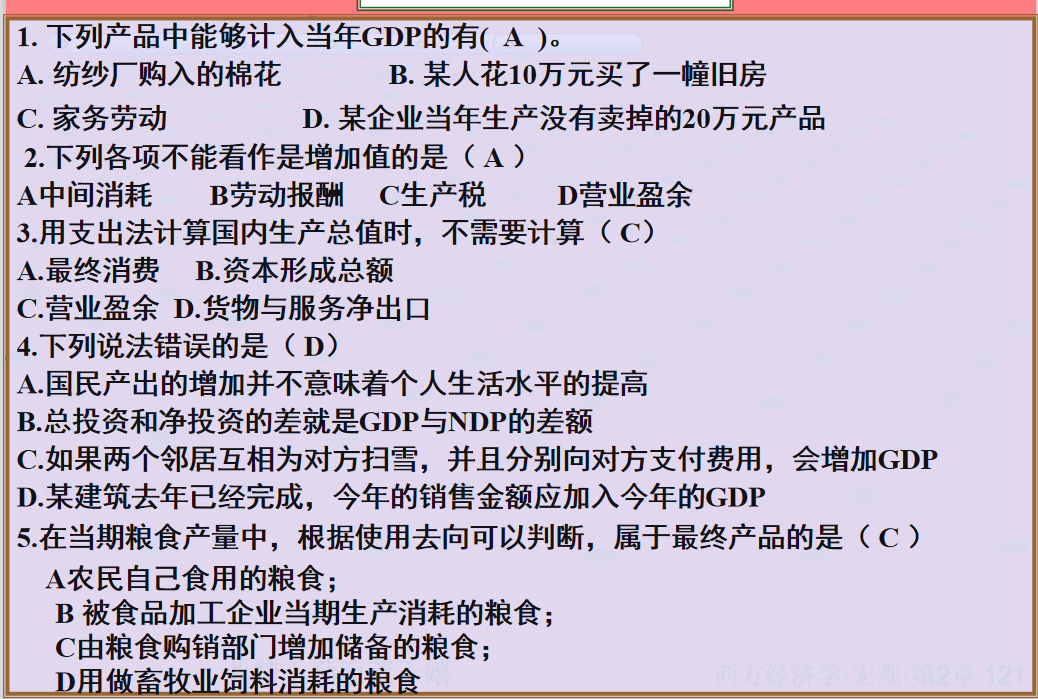最终产品：是一定时期内生产的而在同期内不再加工、可供最终消费和使用的产品。它是从国民经济投入产出平衡的角度考察经济工作成果的重要指标，也是计算国民生产总值的基础。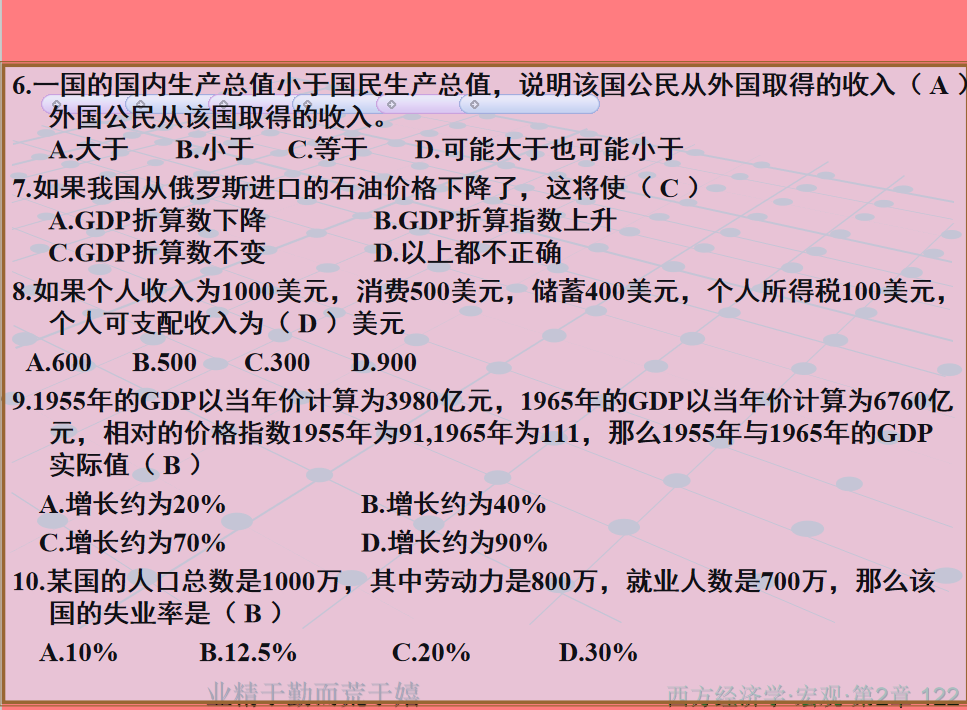7.GDP折算数只包括国内产品价格，不包括进口产品价格，但进口产品价格变动会影响CPI，因为居民也会购买进口消费品；如日本制造并在美国销售的丰田汽车的价格变动，会影响美国的CPI，但不影响美国的GDP折算指数。

GDP折算指数=名义GDP/实际GDP

8.消费+存储

9.(6760/111)/(3980/91)-1=0.39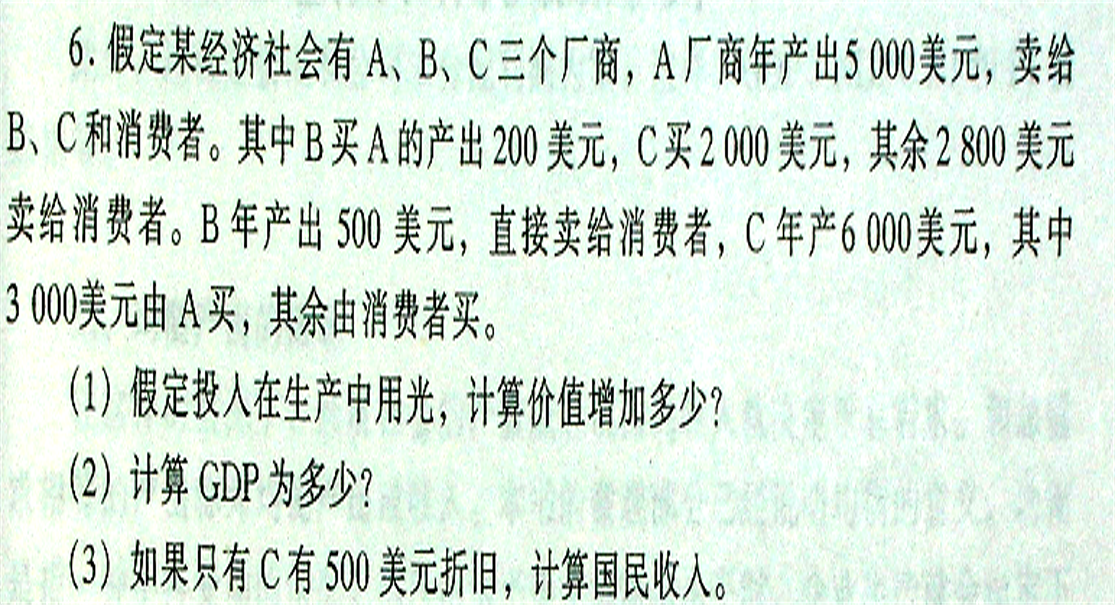1. 5000-3000+500-200+6000-2000=6300

2.2800+500+3000=6300

3.6300-500=5800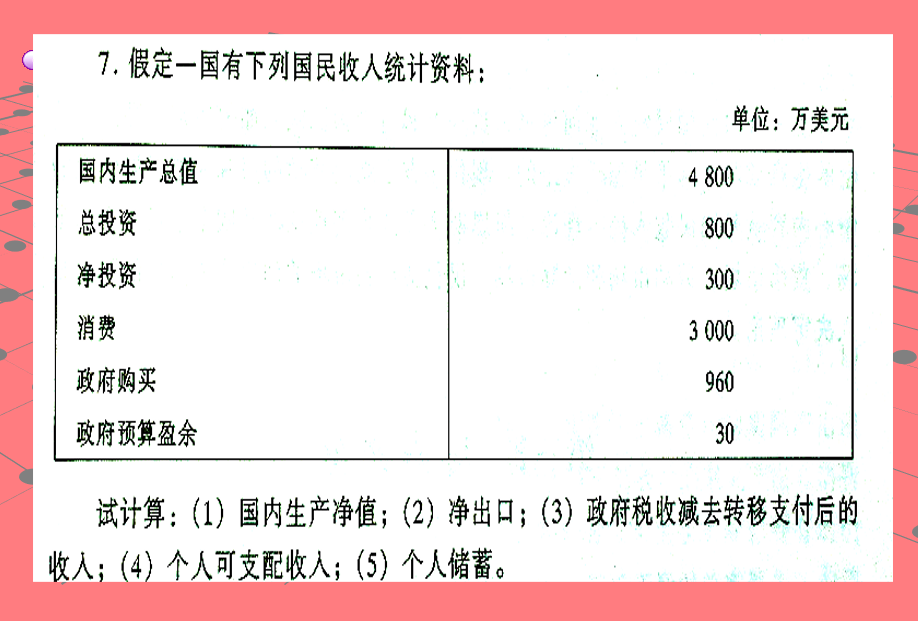1.4800-(800-300)=4300

2.4800-800-3000-960=40

3.设为T。政府预算盈余=T-G，从而T=30+960=990

4.DI=NNP-T=4300-990=3310

5.S=DI-C=3310-3000=310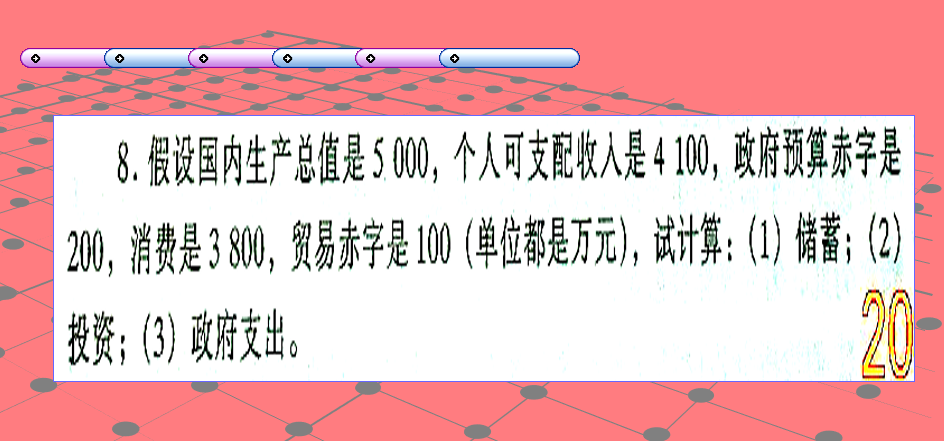支出法：Y=C+I+G+(X-M) 这是支出法计算的GDP

收入法：Y=C+S+T 从收入的支出用途的角度考察的，在三部门中，国民收入要么用在消费C上，要么用在投资I，要么就纳税给  ZF了。

1. 储蓄：4100-3800=300

2.投资 I =S+(T-G)-(X-M)

=300-200
=100

=100+100
=200

3.Y=C+I+G+(X-M)

G=Y-C-I-(X-M)

=5000-3800-200+100=3100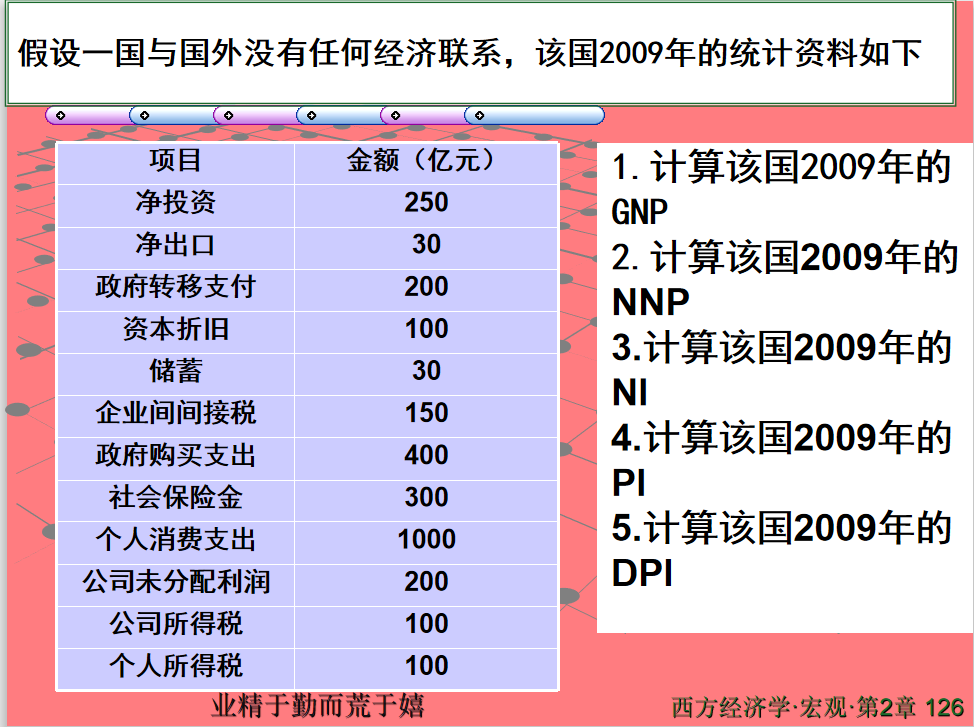GNP=消费+总投资（投资+折旧）+政府购买+进出口=1000+400+100+250+30=1780

NNP（国民生产净值）=GNP-资本折旧=1680

NI（国民收入）= NNP-企业间接税收=1680-150=1530

PI（个人收入）=NI-公司所得税-公司为分配利润-社会保障金+政府转移支付=1530-100-200-300+200=1130

DPI（个人可支配利润）=PI-个人所得税=1130-100=1030